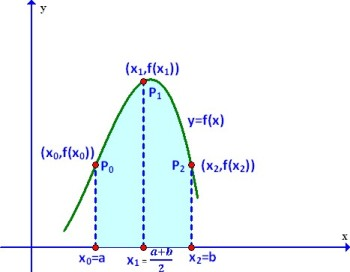# Simpson’s 1/3 Rule – C Program

Simpson’s Rule is a Numerical technique to find the definite integral of a function within a given interval.

The function is divided into many sub-intervals and each interval is approximated by a quadratic curve. And the area is then calculated to find the integral. The more is the number of sub-intervals used, the better is the approximation.
NOTE: The no. of sub-intervals should be EVEN.### Formula Used:$\int_a^bf(x)dx \approx \frac{h}{3}(f(a)+4f(a+h)+2f(a+2h)+4f(a+3h)+2f(a+4h)+....+f(b))$$\implies \int_a^bf(x)dx \approx \frac{h}{3}(f(a)+4f(x_1)+2f(x_2)+4f(x_3)+2f(x_4)+...+f(b))$

where$x_i=a+ih$ for$i=1,2,....n-1$ and$h=(b-a)/n$
The following C Program uses the Simpson’s 1/3 Rule to find the definite integral of a function.
Users, will have to change the function f in the following program to the function whose integral they want to find.

### PROGRAM (Simple Version):

/*********************************
*******SIMPSON'S 1/3 RULE********
2017 (c) Manas Sharma - https://bragitoff.com
********************************/
#include<stdio.h>
#include<math.h>

/* Define the function to be integrated here: */
double f(double x){
return x*x;
}

/*Program begins*/
main(){
int n,i;
double a,b,h,x,sum=0,integral;
/*Ask the user for necessary input */
printf("\nEnter the no. of sub-intervals(EVEN): ");
scanf("%d",&n);
printf("\nEnter the initial limit: ");
scanf("%lf",&a);
printf("\nEnter the final limit: ");
scanf("%lf",&b);
/*Begin Simpson's Procedure: */
h=fabs(b-a)/n;
for(i=1;i<n;i++){
x=a+i*h;
if(i%2==0){
sum=sum+2*f(x);
}
else{
sum=sum+4*f(x);
}
}
integral=(h/3)*(f(a)+f(b)+sum);
printf("\nThe integral is: %lf\n",integral);
}



### OUTPUT:

The above program returns a better approximation to the interval as the number of sub-intervals is increased. This might work for some applications, however, sometimes one might not want to deal with the number of sub-intervals, but rather the accuracy upto a certain decimal places. What I mean by accuracy is that sometimes you might just want the approximate value of integral upto a few decimal place. So you will have to keep on increasing the number of sub-intervals and check the value of the integral. If the integral for two subsequent no. of sub-intervals is within the accuracy/tolerance limit given by the user(or set by you) then the integral should be printed out.

The following program illustrates the process of achieving what I just explained and also uses a function called ‘simpsons’ that handles the integration part.

### PROGRAM (Better Version):

/************************************************
*******SIMPSON'S 1/3 RULE USING FUNCTION********
2017 (c) Manas Sharma - https://bragitoff.com
***********************************************/
#include<stdio.h>
#include<math.h>

/* Define the function to be integrated here: */
double f(double x){
return x*x;
}

/*Function definition to perform integration by Simpson's 1/3rd Rule */
double simpsons(double f(double x),double a,double b,int n){
double h,integral,x,sum=0;
int i;
h=fabs(b-a)/n;
for(i=1;i<n;i++){
x=a+i*h;
if(i%2==0){
sum=sum+2*f(x);
}
else{
sum=sum+4*f(x);
}
}
integral=(h/3)*(f(a)+f(b)+sum);
return integral;
}

/*Program begins*/
main(){
int n,i=2;
double a,b,h,x,sum=0,integral,eps,integral_new;

/*Ask the user for necessary input */
printf("\nEnter the initial limit: ");
scanf("%lf",&a);
printf("\nEnter the final limit: ");
scanf("%lf",&b);
printf("\nEnter the desired accuracy: ");
scanf("%lf",&eps);
integral_new=simpsons(f,a,b,i);

/* Perform integration by simpson's 1/3rd for different number of sub-intervals until they converge to the given accuracy:*/
do{
integral=integral_new;
i=i+2;
integral_new=simpsons(f,a,b,i);
}while(fabs(integral_new-integral)>=eps);

printf("\nThe integral is: %lf for %d sub-intervals.\n",integral_new,i);
}


### OUTPUT:[wpedon id="7041" align="center"]